# choice()

## Syntax

```choice(sequence)
```

## Description

Returns one random item from the `sequence` parameter (a tuple, list, or string). The choice() command is syntactic sugar for the comparatively awkward incantation:

```sequence[random(len(sequence)]
```

## Returns

a random item from the given sequence

## Example

```words = ["It is certain", "Reply hazy try again", "Outlook not so good"]
c = choice(words)
# Variable c contains a random item from the words list
# It might now be "It is certain"...
# or "Reply hazy try again"...
# or "Outlook not so good"
```

# files()

## Syntax

```files(pattern, case=True)
```

## Description

Retrieves the names of all files at a given path matching a wildcard pattern. By default a case-sensitive search is performed but this can be relaxed by passing `False` as the `case` parameter.

## Returns

a list with the names of the files matching the given pattern

## Example

```f = files("~/Pictures/*.jpg") # tilde expands to \$HOME
image(choice(f), 10, 10)
```

# fonts()

## Syntax

```fonts(like=None, western=True)
```

## Description

Searches the fonts installed on the system and can filter the list in a few ways. When called with no arguments, the list contains all fonts on the system with a latin character set. To retrieve the set of non-latin fonts, pass `False` as the `western` parameter. To filter the list through a (case-insensitive) font name match, pass a substring as the `like` parameter.

## Returns

a list of font family names which can be used as arguments for the font() command

## Example

```romans = fonts() # Get a list of all the text-friendly families on the system
jenson = fonts(like="jenson") # returns ["Adobe Jenson Pro"]

eastern = fonts(western=False) # all asian-character fonts, math fonts, etc.
kozuka = fonts(western=False, like="koz") # the Kozuka Gothic & Mincho families
```

# grid()

## Syntax

```grid(cols, rows, colsize=1, rowsize=1)
```

## Description

The grid() command returns an iteratable object, something that can be traversed in a for-loop. You can think of it as being quite similar to the range() command. But rather than returning a series of ‘index’ values, grid() returns pairs of ‘row’ and ‘column’ positions.

The first two parameters define the number of columns and rows in the grid. The next two parameters are optional, and set the width and height of one cell in the grid. In each iteration through the for-loop, your counters are updated with the position of the next cell in the grid.

## Returns

tuples of x/y coordinates that can be used in a for-loop

## Example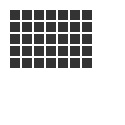```fill(0.2)
for x, y in grid(7, 5, 12, 12):
rect(10+x, 10+y, 10, 10)
```

# measure()

## Syntax

```measure(grob)
measure(image="path", width=None, height=None)
measure("text", width=None, height=None, **fontstyle)
```

## Description

Returns width & height for graphics objects, strings, or images. If the first argument is a string, the size will reflect the current font() settings and will layout the text using the optional `width` and `height` arguments (as well as any keyword arguments supported by the text() command).

If called with an image keyword argument, PlotDevice will expect it to be the path to an image file and will return its pixel dimensions.

If called with a Bezier, returns its ‘bounding box’ dimensions.

## Returns

a Point tuple with the object’s dimensions

# ordered()

## Syntax

```ordered(list, *names, reverse=False)
```

## Description

Creates a sorted copy of a list of objects (similar to Python’s built-in `sorted` function). Lists of dictionaries or objects can be sorted based on a common key or attribute if any `name` strings are provided. If more than one name is present, they will be used (in order) as primary, secondary, tertiary, etc. sorting criteria.

Lists are returned in ascending order unless the `reverse` parameter is `True`.

## Returns

a sorted copy of the original sequence

## Example

```students = [{"name":"Alice", "grade":95},
for s in ordered(students, 'grade', 'name'):
>>> 60 Bob
>>> 88 Carol
>>> 95 Alice
>>> 95 Eve
```

# random()

## Syntax

```random(v1=None, v2=None)
```

## Description

Returns a random number that can be assigned to a variable or a parameter. The random() command is useful for all sorts of operations, from picking colors to setting pen widths.

When no parameters are supplied, returns a floating-point (decimal) number between 0.0 and 1.0 (inclusive). When one parameter is supplied, returns a number between 0 and this parameter. When two parameters are supplied, returns a number between the first and the second parameter.

Note that whether the boundaries are integers or floating point values is significant. See the example code for the differing behaviors that result.

New random values are returned each time the script runs. A particular sequence of random values can be locked-in by supplying a custom random seed:

```from random import seed
seed(0)
```

## Returns

a random number (either an integer or floating-point) within the given boundaries

## Example

```r = random() # returns a float between 0 and 1
r = random(2.5) # returns a float between 0 and 2.5
r = random(-1.0, 1.0) # returns a float between -1.0 and 1.0
r = random(5) # returns an int between 0 and 5
r = random(1, 10) # returns an int between 1 and 10

# sets the fill to anything from
# black (0.0,0,0) to red (1.0,0,0)
fill(random(), 0, 0)
```

## Syntax

```read(path, format=None, encoding='utf-8', cols=None, dict=dict)
```

## Description

Returns the contents of a file located at `path` as a unicode string or format-dependent data type (with special handling for `.json` and `.csv` files).

The format will either be inferred from the file extension or can be set explicitly using the `format` arg. Text will be read using the specified `encoding` or default to UTF-8.

JSON files will be parsed and an appropriate collection type will be selected based on the top-level object defined in the file. The optional keyword argument `dict` can be set to adict or odict if you’d prefer not to use the standard Python dictionary when decoding pairs of `{}`’s.

CSV files will return a list of rows. By default each row will be an ordered list of column values. If the first line of the file defines column names, you can call read() with `cols=True` in which case each row will be a dictionary using those names as keys. If the file doesn’t define its own column names, you can pass a list of strings as the `cols` parameter.

## Returns

a unicode string, list of rows (for CSV files), or Python collection object (for JSON)

## Example

```lipsum = read('lorem.txt')
print lipsum[:11]        # lorem ipsum
print lipsum.split()[:3] # [u'Lorem', u'ipsum', u'dolor']
```
```rows = read('related/months.csv', cols=True)
print rows['english'], rows['days'] # January 31
print rows['french'] # Février
print rows['german'] # März
```
```oracle = read('8ball.json')
prognosis = choice(oracle.keys())
outcome = choice(oracle[prognosis])
print (prognosis, outcome) # (u'neutral', u'Better not tell you now')
```

data: lorem.txt, months.csv, and 8ball.json

# shuffled()

## Syntax

```shuffled(sequence)
```

## Description

Scrambles the order of a sequence without modifying the original. The `sequence` argument can be a list, tuple, or string and the return value will be of the same type.

## Returns

a random permutation of the sequence

## Example

```seq = range(10)
print shuffled(seq) # [7, 4, 8, 3, 9, 2, 0, 5, 6, 1]
print shuffled(seq) # [4, 5, 2, 3, 7, 8, 9, 6, 1, 0]
print shuffled(seq) # [4, 6, 2, 7, 3, 0, 8, 1, 5, 9]
print seq           # [0, 1, 2, 3, 4, 5, 6, 7, 8, 9]
```

# var()

## Syntax

```var(name, type, default, min, max)
```

## Description

The variables sheet creates connections between a variable and a user interface control, such as a slider, textfield or check box. Variables created with the var() command become part of the global namespace, meaning you can access them just like any other variable.

The `name` parameter must be a string, but thereafter can be referred to as a plain variable (without quoting). The variable’s `type` must be `NUMBER`, `TEXT`, `BOOLEAN`, or `BUTTON`. Unless a `default` value is passed, the variable will be initialized to `50` for numbers, `True` for checkboxes, or ‘`hello`’ for text. The `min` and `max` set the range for number-type variables and default to the range 0–100.

## Example```var("amount", NUMBER, 30, 0, 100)

background(0.1, 0, 0.0)
for i in range(int(amount)):
rotate(15)
image("10.png", 5, 5, alpha=0.25)
```

# dictionaries

Python’s built-in dictionary type has the advantages of being fast, versatile, and standard. But sometimes you’ll come across situations where you wish it worked slightly differently. PlotDevice includes a handful of dict-like classes to make a few common access patterns easier or less verbose.

## Attribute DictionaryDerived from the web.py project’s Storage class.

The `adict()` command creates a dictionary whose items may also be accessed with dot notation. Items can be assigned using dot notation even if a dictionary method of the same name exists. Subsequently, dot notation will still reference the method, but the assigned value can be read out using traditional `d["name"]` syntax.

```d = adict(torches=12, rations=3, potions=0)
d.keys = 9
print d.rations # 3
print d.keys()  # ['keys', 'torches', 'potions', 'rations']
print d['keys'] # 9
```

## Default DictionaryA thin wrapper for collections.defaultdict

The `ddict()` command creates a dictionary with a default ‘factory’ function for lazily initializing items. When your code accesses a previously undefined key in the dictionary, the factory function is run and its return value is assigned to the specified key.

```normal_dict = {}
normal_dict['foo'].append(42) # raises a KeyError

lst_dict = ddict(list)
lst_dict['foo'].append(42)    # sets 'foo' to 

num_dict = ddict(int)
print num_dict['bar']         # prints '0'
num_dict['baz'] += 2          # increments 'baz' from 0 to 2

nest_dict = ddict(dict)
nest_dict['apple']['color'] = 'green'
print nset_dict               # prints ddict{'apple': {'color': 'green'}}
```

## Ordered DictionaryA thin wrapper for collections.OrderedDict

the `odict()` command creates a dictionary that remembers insertion order. When you iterate using its keys(), values(), or items() methods, the values will be returned in the order they were created (or, e.g., deserialized from JSON).

Standard `dict` objects return keys in an arbitrary order. This poses a problem for initializing an `odict` since both `dict`-literals and keyword arguments will discard the ordering before the `odict` constructor can access them.

To initialize an `odict` and not lose the key-order in the process, pass a list of (key,val) tuples:

```odict([ ('foo',12), ('bar',14), ('baz', 33) ])
```

or construct it as part of a generator expression:

```odict( (k,other[k]) for k in ordered(other.keys()) )
```

# Point

## Syntax

```Point(x, y)
```

## Description

A pair of x & y coordinates wrapped into a single value. You can access its properties by name in addition to using the object as a ‘normal’ tuple. Point objects support basic arithmetic and provide utility methods for geometry calculations.

PlotDevice commands that take separate x & y arguments (e.g., poly(), arc(), text()) will typically also accept a Point object in their place.

```pt.x
pt.y
```

## Methods

```pt.angle(point)
```

Returns the angle from the object to a target Point or pair of (x,y) coordinates. By default, the angle will be represented in degrees, but this can be altered by setting an alternate unit with the geometry() command.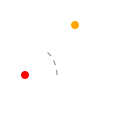```src = Point(25,75)
dst = Point(75,25)
theta = src.angle(dst)
arc(src, 4, fill='red')
arc(dst, 4, fill='orange')
with pen(dash=5), stroke(.5), nofill():
arc(src, 32, range=theta)
print theta
>>> -45.0
```
```pt.distance(point)
```

Returns the linear distance between the object and a second Point or pair of (x,y) coordinates.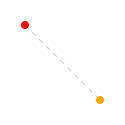```src = Point(25,25)
dst = Point(100,100)
length = src.distance(dst)
arc(src, 4, fill='red')
arc(dst, 4, fill='orange')
with pen(dash=5), stroke(.8):
line(src, dst)
print length
>>> 106.066017178
```
```pt.reflect(point, d=1.0, a=180)
```

Uses the object as an ‘origin’ and returns a Point containing the reflection of a target Point or pair of (x,y) coordinates. The optional `d` parameter is a magnification factor relative to the distance between the mirror and the target point.

The `a` parameter controls the angle of reflection. When set to 180° (the default), the reflected point will be directly opposite the target, but other values will allow you to ‘ricochet’ the target point off of the mirror instead.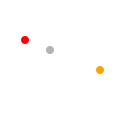```origin = Point(50,50)
src = Point(25,40)
dst = origin.reflect(src, d=2.0)
arc(src, 4, fill='red')
arc(dst, 4, fill='orange')
arc(origin, 4, fill=.7)
```
```pt.coordinates(distance, angle)
```

Uses the object as the ‘origin’ of a polar coordinate system and returns a Point at a given distance and orientation relative to the origin. The `distance` parameter uses the current canvas size() unit and the `angle` should be expressed in the current geometry() unit.```origin = Point(50,50)
dst = origin.coordinates(50, 45)
arc(origin, 4, fill=.7)
arc(dst, 4, fill='orange')
with pen(dash=3), stroke(.5), nofill():
arc(origin, 25, range=45)
```

If you’d prefer to work with raw numbers rather than Point objects, you can also import the numerical versions of these methods for use in your scripts:

```from plotdevice.lib.pathmatics import angle, distance, coordinates, reflect
```

Geometry

# Region

## Syntax

```Region(x, y, w, h)
Region(Point, Size)
```

## Description

An origin Point and a Size which together describe a rectangular region. Region objects have properties to read and modify the rectangle geometry. Methods allow you to generate new regions from combinations of old ones or by systematically shifting their values.

PlotDevice commands that take `x`/`y`/`w`/`h` arguments (e.g., rect(), oval(), image()) will also accept a Region object in their place.

## Geometry Properties

```r.x, r.y # position
r.w, r.h # dimensions
r.origin # Point(r.x, r.y)
r.size   # Size(r.w, r.h)
```

## Convenience Properties

```r.top, r.bottom, r.left, r.right # edge positions
r.w/h/t/b/l/r # shorthand aliases
```

## Methods

```r.intersect(region)
```

return a new Region describing the overlapping portions of the existing Region object and a second Region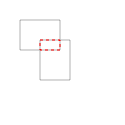```r1 = Region(20,20, 40,30)
r2 = Region(40,40, 30,40)
overlap = r1.intersect(r2)
with nofill(), stroke(.7):
rect(r1)
rect(r2)
rect(overlap, stroke='red', dash=3)
```
```r.union(region)
```

return a new Region that contains the combined bounds of the existing Region object and a second Region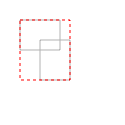```r1 = Region(20,20, 40,30)
r2 = Region(40,40, 30,40)
union = r1.union(r2)
with nofill(), stroke(.7):
rect(r1)
rect(r2)
rect(union, stroke='red', dash=3)
```
```r.shift(point)
```

return a copy of the Region with its origin shifted by a Point```r = Region(20,20, 40,30)
shifted = r.shift(20,15)
nofill()
rect(r, stroke=.7)
rect(shifted, stroke='red', dash=3)
```
```r.inset(dx, dy)
```

return a copy of the Region whose horizontal dimensions have been shrunk by `dx` and vertical by `dy`. If `dy` is omitted, shrink both dimensions by `dx`. Passing a negative value for `dx` or `dy` will enlarge the dimension rather than shrinking it.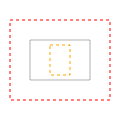```r = Region(30,40, 60,40)
shrunk = r.inset(20,5)
grown = r.inset(-20)
nofill()
rect(r, stroke=.7)
rect(shrunk, stroke='orange', dash=3)
rect(grown, stroke='red', dash=3)
```

Geometry

# Size

## Syntax

```Size(width, height)
```

## Description

A pair of width & height values wrapped into a single object. Size objects are very similar to Points and arithmetic between the two types is supported.

## Properties

```s.width
s.height
s.w, s.h # shorthand aliases
```

Geometry

# autotext()

## Syntax

```autotext()
```

## Description

Generates random text from an XML-document. Autotext is a very powerful feature once you learn how to use it. PlotDevice has a built-in implementation of the Kant Generator Pro. XML-files for the Kant Generator have a specified structure. Further information can be found at Dive into Python’s chapter on XML Processing.

## Returns

a string containing automatically generated text

## Example```fontsize(9)
lineheight(0.8)
txt = autotext("kant.xml")
text(txt, 5, 10, width=110)
```

# imagesize()

## Syntax

```imagesize(path)
```

## Description

Returns the dimensions (width and height) of an image located at the given path. Obtaining the size of an image is useful when the image needs to scaled to an exact size, for example. The return value is a list with the image’s width and the image’s height.

Note that these dimensions may also be retrieved from the `size` property of the Image object returned by the image() command.

## Returns

a tuple with the width and height of the given image

## PlotDevice Equivalent

The width & height of any graphical object (including Images) can be retrieved using the measure() command.

## Example

```w, h = imagesize("superfolia.jpg")
print w
print h
```

# open()

## Syntax

```open(path).read()
```

## Description

The open() command opens a file specified by the path parameter. The open() command can be used in two ways: open(path).read(), which returns the file’s text content as a string, or, alternatively, open(path).readlines(), which returns a list of text lines.

Note that open() is actually a part of the Python language. See the official docs for details on using open() and dealing with the file objects it returns.

## Returns

a file object whose `read` method provides its contents as a string

## PlotDevice Equivalent

A more convenient way to read the contents of a file into a string (or other data structure) is the new read() command. It can handle text files as well as CSV- or JSON-formatted data.

## Example

```# Prints the contents of sample.txt as a whole